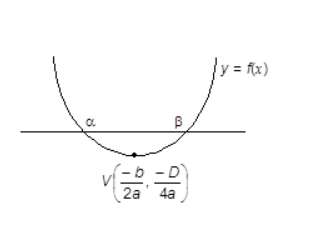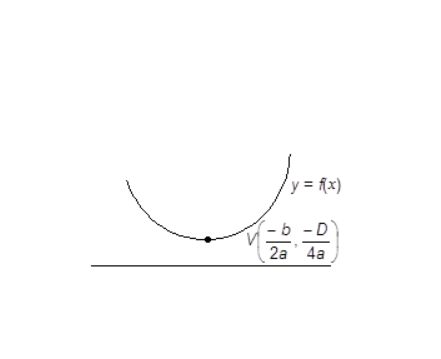## Filters

Sort by :
Clear All
Q
Engineering
30 Views   |

LetwhereThenequals :

• Option 1)• Option 2)• Option 3)• Option 4)Option 2

Engineering
56 Views   |

If (10)9 + 2(11)1   (10)8 + 3(11)2  (10)7 +......  +10 (11)9 = k (10)9, then k is equal to :

• Option 1)

100

• Option 2)

110

• Option 3)• Option 4)Use

Sum of n terms of a GP -

- wherein

first term

common ratio

number of terms

and

(10)9 + 2(11)1 (10)8 + 3(11)2 (10)7 +......  +10 (11)9 = k(10)9

Take common 109

Subtract

Option 1)

100

Option 2)

110

Option 3)Option 4)View More
Engineering
319 Views   |

If the coefficients of x3 and x4 in the expansion of (1 + ax + bx2) (1 - 2x)18 in powers of x are both zero, then (a, b) is equal to :

• Option 1)• Option 2)• Option 3)• Option 4)As we have learned Expression of Binomial Theorem -   - wherein for n  +ve integral .     coeff of   coeff of    and  and   a = 16  b = 272/3        Option 1) Option 2) Option 3) Option 4)
Engineering
282 Views   |

The sum of first 20 terms of the sequence 0.7 ,0.77,0.777,.........,is :

• Option 1)• Option 2)• Option 3)• Option 4)As we learnt

Sum of infinite terms of a GP -

- wherein

first term

common ratio

Option 1)Option 2)Option 3)Option 4)View More
Engineering
134 Views   |

The graph of the function    is symmetrical about the line ,then

• Option 1)

• Option 2)

• Option 3)

• Option 4)

As we learnt in

Even Function -

- wherein

Since a graph symmetric about y-axis

means  x = 0 then it is even function and f(-x) = f(x)

f(0 - x) = f(0 + x)     (b < z it is symmetric about v = 0 )

But in question it is symmetric about x = 2

then f(x - 2) = f(x + 2)

Correct option is 3.

Option 1)

Option 2)

Option 3)

Option 4)

View More
Engineering
133 Views   |

ifand• Option 1)

is an empty set.

• Option 2)

contains exactly one element.

• Option 3)

contains exactly two elements.

• Option 4)

contains more than two elements.

As we learnt in

FUNCTIONS -

A relation f from a set A to a set B is said to be a function if every element of set A has one and only one image in set B.

-

Put  at the place of

(i)

(ii)

Multiplying (i) by 2

and

Correct option is 3.

Option 1)

is an empty set.

Option 2)

contains exactly one element.

Option 3)

contains exactly two elements.

Option 4)

contains more than two elements.

View More
Engineering
124 Views   |

The real numberfor which the equation,   has two distinct real roots in• Option 1)

does not exist.

• Option 2)

lies between 1 and 2 .

• Option 3)

lies between 2 and 3 .

• Option 4)

lies between -1 and 0 .

As we have learned

Quadratic Expression Graph when a> 0 & D > 0 -

Real and distinct roots of

&

- whereinis the abscissa of vertex

and , it should lie in(0,1 ) but it's not true

S, no value of 'k' exists

Option 1)

does not exist.

Option 2)

lies between 1 and 2 .

Option 3)

lies between 2 and 3 .

Option 4)

lies between -1 and 0 .

View More
Engineering
124 Views   |

Letandbe the roots of equationare in A.P. andthen the value ofis ?

• Option 1)• Option 2)• Option 3)• Option 4)As we have learned

Sum of Roots in Quadratic Equation -

- wherein

Product of Roots in Quadratic Equation -

- wherein

@1449

Also

Also p+r =2q

Option 1)Option 2)Option 3)Option 4)View More
Engineering
133 Views   |

If z is a complex number of unit modulus and argument,then argequals:

• Option 1)• Option 2)• Option 3)• Option 4)As we have learned

Euler's Form of a Complex number -

- wherein

r denotes modulus of z and  denotes argument of z.

Polar Form of a Complex Number -

- wherein

r= modulus of z and  is the argument of zArg (z)=

So,

Option 1)Option 2)Option 3)Option 4)View More
Engineering
135 Views   |

If the equationshave a common root, then a : b : c is :

• Option 1)

3 : 1 : 2

• Option 2)

1 : 2 : 3

• Option 3)

3 : 2 : 1

• Option 4)

1 : 3 : 2

As we have learned

Quadratic Expression Graph when a > 0 & D < 0 -

No Real and Equal root of

&

- whereinCondition for both roots common -

- wherein

&

are the 2 equations

For

Discriminant = 4-12 = -8 < 0

Both the roots are common as complex roots occur in conjugate

Option 1)

3 : 1 : 2

Option 2)

1 : 2 : 3

Option 3)

3 : 2 : 1

Option 4)

1 : 3 : 2

View More
Engineering
105 Views   |

Ifis the adjoint of a 3 x 3  matrix A and= 4,thenis equal to :

• Option 1)

0

• Option 2)

4

• Option 3)

11

• Option 4)

5

As we have learned

Property of adjoint of A -

- wherein

denotes adjoint of  and    denotes determinant  of  and  is the order of the matrix

Option 1)

0

Option 2)

4

Option 3)

11

Option 4)

5

View More
Exams
Articles
Questions